# Common Core: High School - Geometry : Prove Laws of Sines and Cosines: CCSS.Math.Content.HSG-SRT.D.10

## Example Questions

### Example Question #1 : Prove Laws Of Sines And Cosines: Ccss.Math.Content.Hsg Srt.D.10

The Law of Cosines is a generalization of which common theorem?

Triangle Proportionality Theorem

Triangle Sum Theorem

Pythagorean Theorem

Fundamental Theorem of Algebra

Side Angle Side Similarity Theorem

Pythagorean Theorem

Explanation:

The Law of Cosines is a generalization of the Pythagorean Theorem. To better understand this, start by drawing triangle ABC with sides a, b, and c. Then, construct the altitude from point A to get height h. Finally label the distance between point B and the point where h meets side a as distance r.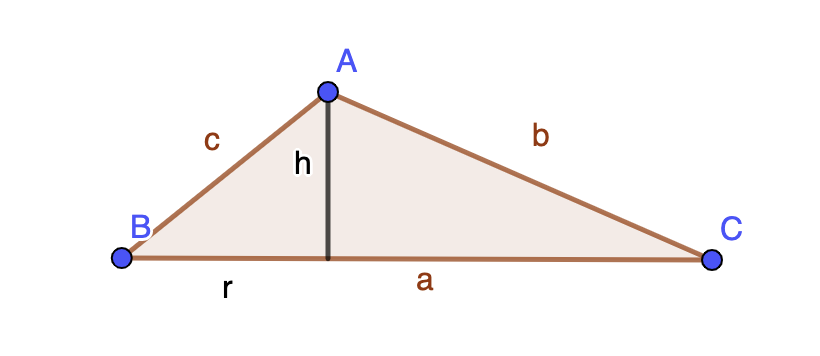We will arbitrarily work to solve for side b, but we could do the same for a and c (after drawing the correct altitudes), which is why we can generalize that the other two formulas to find a and c follow.

By definition, we know that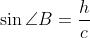. If we multiply both sides by c, we get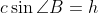.

Also, we know that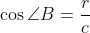. If we multiply both sides by c, we get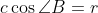.

Using the Pythagorean Theorem, we get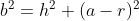.

Substituting in h and r from above, we get: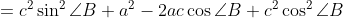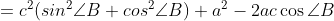(because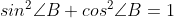)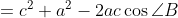Therefore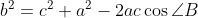.

The other two versions of Law of Cosines are: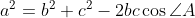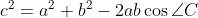### Example Question #1 : Prove Laws Of Sines And Cosines: Ccss.Math.Content.Hsg Srt.D.10

True or false: The Law of Sines applies to all types of triangles.

False

True

True

Explanation:

This is true. While the Pythagorean Theorem only applies to right triangles, the Law of Sines applies to all triangles no matter if they are right, acute, or obtuse.

### Example Question #1 : Prove Laws Of Sines And Cosines: Ccss.Math.Content.Hsg Srt.D.10

True or False: The Law of Cosines applies to all triangles.

True

False

True

Explanation:

This is true. While the Pythagorean Theorem only applies to right triangles, the Law of Cosines applies to all triangles no matter if they are right, acute, or obtuse.

### Example Question #2 : Prove Laws Of Sines And Cosines: Ccss.Math.Content.Hsg Srt.D.10

Choose the answer choice that correctly states the Law of Sines, given the triangle below.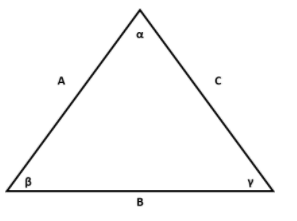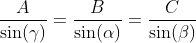Explanation:

The Law of Sines uses ratios of a triangle’s sides and angles to allow us to be able to solve for unknown sides and/or angles.  This relationship is true for any triangle.The Law of Sines is.

### Example Question #3 : Prove Laws Of Sines And Cosines: Ccss.Math.Content.Hsg Srt.D.10

Which of the following expressions correctly states the Law of Cosines? Use the following triangle for reference.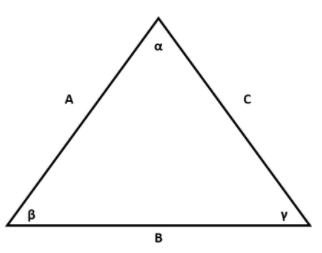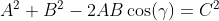Explanation:

The Law of Cosines should look similar to a theorem we use quite commonly for right triangles.  The Law of Cosines is basically the Pythagorean Theorem, but it has an added argument so that it is true for any and all triangles.The Law of Cosines is### Example Question #1 : Prove Laws Of Sines And Cosines: Ccss.Math.Content.Hsg Srt.D.10

Choose the answer choice that correctly states the Law of Sines, given the triangle below.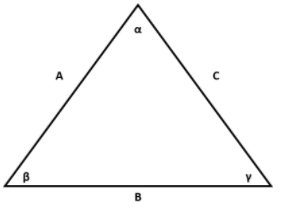Explanation:

The Law of Sines uses ratios of a triangle’s sides and angles to allow us to be able to solve for unknown sides and/or angles.  This relationship is true for any triangle.The Law of Sines is.

### All Common Core: High School - Geometry Resources